# The Photoelectric Effect

This situation changed in 1905 when Einstein extended the photon picture to explain another phenomenon of light which could not be accounted for in the conventional wave picture - the photoelectric effect. In this effect light is shone on a metal, and electrons are released. As indicated in Fig. 27.3, these electrons can be attracted towards a positively charged plate a certain distance below, thereby establishing a photoelectric current.It is convenient not to measure this current itself but to measure the stopping potential V0 required to reduce this current to zero. The stopping potential is related to the (maximum) kinetic energy of the ejected electrons by

 eV0 = KE max =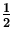mv max2. (4)

There were several failings of the wave picture of light when applied to this phenomenon, but the most notable was the following:
No photoelectric electrons are emitted if the frequency of the light falls below some cutoff frequency, fc .
This aspect of the photoelectric effect is impossible to understand within the wave picture of light, as within that picture the energy of the light beam which gives the electrons their energy does not depend on the frequency.

Einstein came up with an explanation of the photoelectric effect which built upon Planck's photon hypothesis. In this theory Einstein assumed that photons have an energy equal to the energy difference between adjacent levels of a blackbody:

 E = hf. (5)

When these photons hit the metal, they could give up some or all of their energy to an electron. A certain amount of energy would be required to release the electrons from their bonds to the metal - this energy is called the work function,, of the metal. The remaining energy would appear as kinetic energy of the released electron. Thus, the maximum kinetic energy the electrons could have is

 KE max = eV0 = hf -=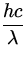-. (6)

Thus, a graph such as in Fig. 27.4 of the stopping potential V0 vs. the frequency f is a straight line, with slope related to Planck's constant h and x -intercept being the cutoff frequency, fc where V0 = 0 :

 fc =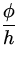=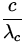, (7)

whereis the corresponding cutoff wavelength. This behaviour is observed experimentally, and the success of this explanation made more and more people take the photon picture of light seriously.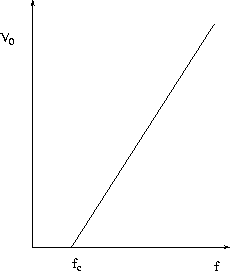Next: Compton Scattering Up: Quantum Physics Previous: Blackbody Radiation